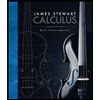# 2. The theorem Existence of Extrema on a Closed Interval guarantees that a continuous function on a closed interval takes on both a minimum and a maximum value on the interval. In such conditions those extrema values occur at critical points or endpoints of the interval. Given the function 12 x - 2, if - 4x < 6 5+6x- 2, f(x) if 6 x 8 TC a. Graph f(x) and explain why it does not fulfill the assumptions of the theorem Existence of Extrema on a Closed Interval on -4, 8 Will the extrema still exist? b. Find the critical points of f(x), then compare the values of f(x) at the critical points and the endpoints of -4, 8. What are the largest and smallest of these values? Is the smallest value found in b. the absolute minimum of f(x)? (Hint: compare with f(5.5)) d. Does f(x) have both extrema on -4, 8]? Why or why not? No it der nat

Question

Problem 2 part A, B, and C

Expert Solution

### Want to see the full answer?

Check out a sample Q&A hereStudents who’ve seen this question also like:Calculus: Early Transcendentals
8th Edition
ISBN: 9781285741550
Author: James Stewart
Publisher: Cengage Learning